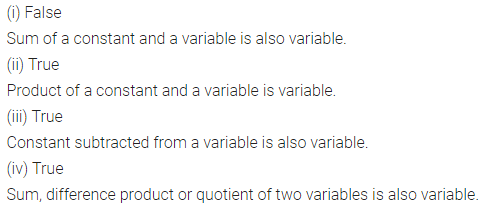# Selina Concise Mathematics Class 6 ICSE Solutions Chapter 18 Fundamental Concepts

## Selina Concise Mathematics Class 6 ICSE Solutions Chapter 18 Fundamental Concepts

Selina Publishers Concise Mathematics Class 6 ICSE Solutions Chapter 18 Fundamental Concepts

### Fundamental Concepts Exercise 18A – Selina Concise Mathematics Class 6 ICSE Solutions

Question 1.
Express each of the following statements in algebraic form :
(i) The sum of 8 and x is equal to y.
(ii) x decreased by 5 is equal to y.
(iii) The sum of 2 and x is greater than y.
(iv) The sum of x and y is less than 24.
(v) 15 multiplied by m gives 3n.
(vi) Product of 8 and y is equal to 3x.
(vii) 30 divided by b is equal to p.
(viii) z decreased by 3x is equal to y.
(ix) 12 times of x is equal to 5z.
(x) 12 times of x is greater than 5z.
(xi) 12 times of x is less than 5z.
(xii) 3z subtracted from 45 is equal to y.
(xiii) 8x divided by y is equal to 2z.
(xiv) 7y subtracted from 5x gives 8z.
(xv) 7y decreased by 5x gives 8z.
Solution:Question 2.
For each of the following algebraic expressions, write a suitable statement in words:
(i) 3x + 8=15
(ii) 7 – y > x
(iii) 2y – x < 12
(iv) 5 ÷ z = 5
(v) a + 2b > 18
(vi) 2x – 3y= 16
(vii) 3a – 4b > 14
(viii) b + 7a < 21
(ix) (16 + 2a) – x > 25
(x) (3x + 12) – y < 3a
Solution:### Fundamental Concepts Exercise 18B – Selina Concise Mathematics Class 6 ICSE Solutions

Question 1.
Separate the constants and the variables from each of the following: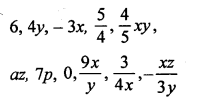Solution: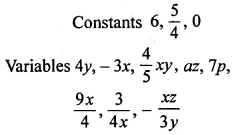Question 2.
Group the like terms together :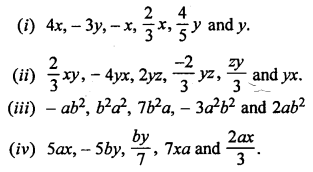Solution: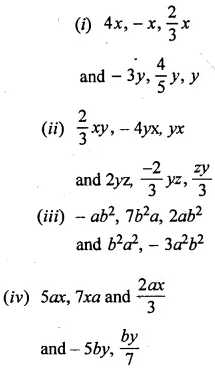Question 3.
State whether true or false :
(i) 16 is a constant and y is a variable but 16y is variable.
(ii) 5x has two terms 5 and x.
(iii) The expression 5 + x has two terms 5 and x
(iv) The expression 2x2 + x is a trinomial.
(v) ax2 + bx + c is a trinomial.
(vi) 8 x ab is a binomial.
(vii) 8 + ab is a binomial.
(viii) x3 – 5xy + 6x + 7 is a polynomial.
(ix) x3 – 5xy + 6x + 7 is a multinomial.
(x) The coefficient of x in 5x is 5x.
(xi) The coefficient of ab in – ab is – 1.
(xii) The coefficient of y in – 3xy is – 3
Solution: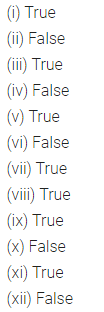Question 4.
State the number of terms in each of the following expressions :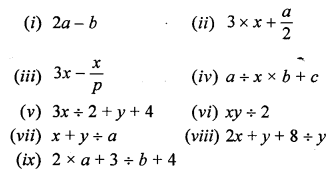Solution: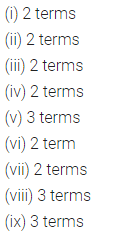Question 5.
State whether true or false:
(i) xy and – yx are like terms.
(ii) x2y and – y2x are like terms.
(iii) a and – a are like terms.
(iv) – ba and 2ab are unlike terms.
(v) 5 and 5x are like terms.
(vi) 3xy and 4xyz are unlike terms.
Solution:Question 6.
For each expression, given below, state whether it is a monomial, or a binomial or a trinomial.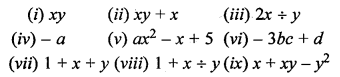Solution: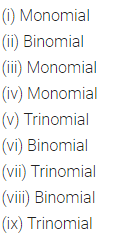Question 7.
Write down the coefficient of x in the following monomial :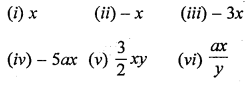Solution:Question 8.
Write the coefficient of :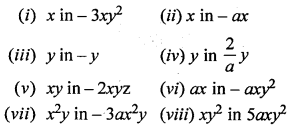Solution: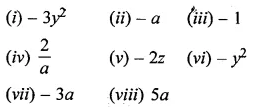Question 9.
State the numeral coefficient of the following monomials :(vii) – 7x ÷ y
(viii) – 3x ÷ (2y)
Solution: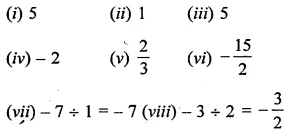Question 10.
Write the degree of each of the following polynomials :Solution:### Fundamental Concepts Revision Exercise – Selina Concise Mathematics Class 6 ICSE Solutions

Question 1.
Express each of the following statements in algebraic form :
(i) The sum of 3x and 4y is 8.
(ii) 5x decreased by 7 gives y.
(iii) 31 added to 4x gives 6x.
(iv) 3x subtracted from 89 gives 44.
Solution:Question 2.
Group the like terms :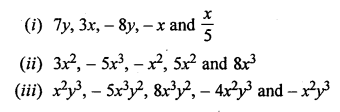Solution:Question 3.
Write the number of terms in each of the following polynomials :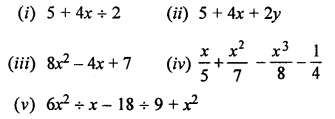Solution: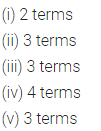Question 4.
For each expression, given below, state whether it is a monomial, or a binomial or a trinomial:
(i) x + y
(ii) 5x – 4y
(iii) 7x2 + 5x + 8
(iv) 64 + 3 ÷ 6
(v) 9 ÷ a x b
(vi) 8a ÷ b
Solution:Question 5.
Write the coefficient of x2y in :
(i) -7x2yz
(ii) 8abx2y
(iii) – x2y
Solution: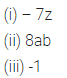Question 6.
Write the coefficient of :
(i) x2 in – 8x2y
(ii) y in -4y
(iii) x in – xy2
Solution: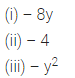Question 7.
Write the numeral coefficient in :Solution:Question 8.
Write the degree of each of the following polynomials :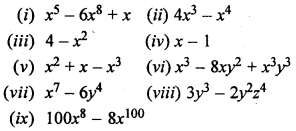Solution: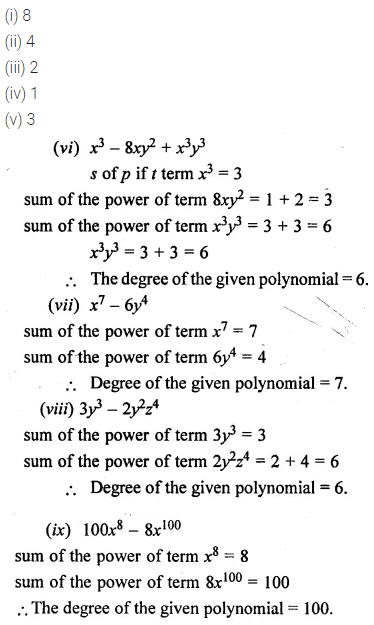Question 9.
Write each statement, given below in algebraic form :
(i) 28 more than twice of x is equal to 45.
(ii) 3y reduced by 5z is greater than 8x.
(iii) 6x divided by 13y is less than 17.
(iv) 9 multiplied by 5x is equal to 2y.
Solution: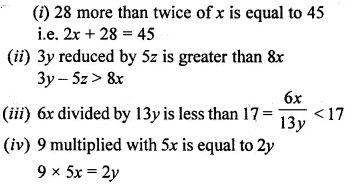Question 10.
State whether true or false :
(i) If 23 is a constant and x is a variable, 23 + x is constant.
(ii) If 23 is a constant and x is a variable, 23x is a variable.
(iii) If y is a variable and 57 is a constant, y – 57 is a variable.
(iv) If 3x and 2y are variable; each of 3x + 2y, 3x – 2y, 3x ÷ 2y and 3x x 2y is a variable.
Solution: